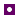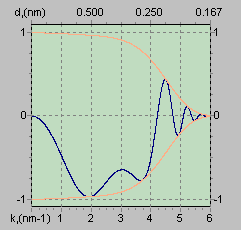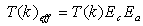Science Behind ItHome Effect of Defocus Effect of Cs Effect of High Voltage Envelope Functions Point Resolution Information Limit Spatial Coherency Passbands (Phase) Contrast Transfer Function or CTF is frequently referred to in (HR)TEM. To put it simple, CTF allows to evaluate and compare performances (point-to-point resolution and information limit) of different microscopes.

CTF (Contrast Transfer Function) is the function which modulates the amplitudes and phases of the electron diffraction pattern formed in the back focal plane of the objective lens. It can be represented as:Clearly, it will be a complicated curve which will depend on:Cs (the quality of objective lens defined by spherical aberration coefficient)l (wave-length defined by accelerating voltage)D f (the defocus value)k (spatial frequency)

The figures below show typical CTF plotted for an imaginary 200 keV microscope:CTF for a 200 keV microscope at "extended" Scherzer defocus. (Envelope functions ARE NOT applied).CTF for the same microscope at "extended" Scherzer defocus. (Envelope functions ARE applied).

Important points to notice:CTF is oscillatory: there are "passbands" where it is NOT equal to zero (good "transmittance") and there are "gaps" where it IS equal (or very close to) zero (no "transmittance").When it is negative, positive phase contrast occurs, meaning that atoms will appear dark on a bright background.When it is positive, negative phase contrast occurs, meaning that atoms will appear bright on a dark background.When it is equal to zero, there is no contrast (information transfer) for this spatial frequency.

Other important features:

1. CTF starts at 0 and decreases, then

2. CTF stays almost constant and close to -1 (providing a broad band of good transmittance), then

3. CTF starts to increase, and

4. CTF crosses the k-axis, and then

5. CTF repeatedly crosses the k-axis as k increases.

6. CTF can continue forever but, in reality, it is modified by envelope functions and eventually dies off. Effect of the envelope functions can be represented as:where Ec is the temporal coherency envelope (caused by chromatic aberrations, focal and energy spread , and instabilities in the high tension and objective lens current), and Ea is spatial coherency envelope (caused by the finite incident beam convergence).

Yet more to note:First crossing of the k-axis at "extended" Scherzer defocus corresponds to point-to-point resolution of the microscope.The point where envelope functions damp the CTF to zero corresponds to the information limit of the microscope.

Home ] Effect of Defocus ] Effect of Cs ] Effect of High Voltage ] Envelope Functions ] Point Resolution ] Information Limit ] Spatial Coherency ] Passbands ]